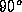Next: Multiple Mother Chirplets: The Up: THE CHIRPLET Previous: Gaussian Chirplet Transform (GCT)

## Continuous Chirplet Transform (CCT)

We have been using the frequency shear operator, which we obtained through multiplication by a linear FM chirp. In a dual manner, we may introduce the time shear operator (Table 1, last row) which we obtain by convolving with a linear FM chirp.

Fourier transformation of a chirp, with chirprate, d, produces another chirp which has chirprate -1/d. Thus convolution of a signal, s(t), with a chirp, having chirprate d is equivalent to multiplying S(f) with a chirp of rate -1/d, and taking the inverse Fourier transform of the product. In short, we have rotated the TF plane, sheared it left-right, and rotated it back. This three-step process has the net effect of shearing the TF plane top-bottom.

The full continuous chirplet transform (CCT) is defined in the same manner as (10):

S_t_c,f_c,(_t),c,d \!=\!

C_t_c,f_c,_t,c,d g(t) \: \: s(t) \!

except that we have one new operator, time-shear, that is composed with the other four operators.

Again, the law of compositionartin of any two chirplet operators (multiplicatively) follows by virtue of the fact that both represent affine coordinate transformations of the TF plane.

The intuition behind (11) is that entries in the first column of Table 1 simply represent the coordinate axes of the multidimensional parameter space, and their subscripts represent the distances along these axes.

Segal exploited various coordinate transformations in the TF plane in the development of his theory of dynamical systems of infinitely many degrees of freedomsegal. His harmonic map or oscillator map, as he called it (the Segal Shale Weil representationshenoyweyl), is indeed related to the chirplet transform.

Steve Mann
Thu Jan 8 19:50:27 EST 1998